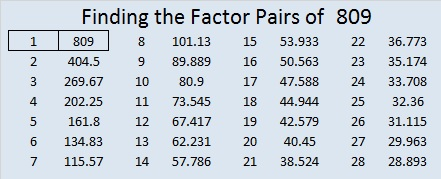# 809 Palindromes, Factors, Whoop-de-dooPrint the puzzles or type the solution on this excel file: 10-factors 807-814

Normally I would tell you that 809 is a palindrome in two different bases:

• 676 in BASE 11 because 6(121) + 7(11) + 6(1) = 809
• 575 in BASE 12 because 5(144) + 7(12) +5(1) = 809

But whoop-de-doo, all that really means is that (x – 11) is a factor of 6x² + 7x – 803, and (x – 12) is a factor of 5x² + 7x – 804.

Isn’t it just as exciting that ⁰¹²³⁴⁵⁶⁷⁸⁹

• (x – 2) is a factor of x⁹+ x⁸ + x⁵ + x³ – 808 because 809 is 1100101001 in BASE 2?
• (x – 3) is a factor of x⁶ + 2x³ + 2x² +2x – 807 because 809 is 1002222 in BASE 3?
• (x – 4) is a factor of 3x⁴ + 2x² + 2x – 808 because 809 is 30221 in BASE 4?
• (x – 5) is a factor of x⁴ + x³ + 2x² + x – 805 because 809 is 11214 in BASE 5?

Notice that the last number in each of those polynomials is divisible by the BASE number.

Palindromes NEVER end in zero so the polynomials they produce will NEVER end in the original base 10 number.

So are palindromes really so special? Today I am much more excited that figuring out what a number is in another base can give us a factor of a corresponding polynomial!

How do I know what those polynomials are? Let me use 809 in BASE 6 as an example:

Since 809 is 3425 in BASE 6, I know that

• 3(6³) + 4(6²) + 2(6¹) + 5(6º) = 809
• 3(216) + 4(36) + 2(6) + 5(1) – 809 = 0
• so 3(216) + 4(36) + 2(6) – 804 = 0
• thus (x – 6) is a factor of 3x³ + 4x² + 2x – 804 because of the factor theorem.

If I told you what 809 is in Bases 7, 8, 9, and 10 would you be able to write the corresponding polynomials that are divisible by (x – 7), (x – 8), (x – 9), and (x – 10) respectively?

• 2234 in BASE 7
• 1451 in BASE 8
• 1088 in BASE 9
• 809 in BASE 10

Scroll down past 809’s factoring information to see if you found the correct polynomials.

—————–

• 809 is a prime number.
• Prime factorization: 809 is prime.
• The exponent of prime number 809 is 1. Adding 1 to that exponent we get (1 + 1) = 2. Therefore 809 has exactly 2 factors.
• Factors of 809: 1, 809
• Factor pairs: 809 = 1 x 809
• 809 has no square factors that allow its square root to be simplified. √809 ≈ 28.4429253066558.How do we know that 809 is a prime number? If 809 were not a prime number, then it would be divisible by at least one prime number less than or equal to √809 ≈ 28.4. Since 809 cannot be divided evenly by 2, 3, 5, 7, 11, 13, 17, 19, or 23, we know that 809 is a prime number.

Here’s another way we know that 809 is a prime number: Since its last two digits divided by 4 leave a remainder of 1, and 28² + 5² = 809 with 28 and 5 having no common prime factors, 809 will be prime unless it is divisible by a prime number Pythagorean triple hypotenuse less than or equal to √809 ≈ 28.4. Since 809 is not divisible by 5, 13, or 17, we know that 809 is a prime number.

——————–

Were you able to find those polynomials from knowing what 809 is in other bases? Check your work with the answers below:

• 2234 Base 7 tells us (x – 7) is a factor of 2x³ + 2x² + 3x – 805
• 1451 Base 8 tells us (x – 8) is a factor of x³ + 4x² + 5x – 808
• 1088 Base 9 tells us (x – 9) is a factor of x³ + 8x – 801
• 809 Base 10 tells us (x – 10) is a factor of 8x² – 800

If you’ve made it this far, even if I’ve made you feel a little dizzy, you’ve done GREAT! Keep up the good work!This site uses Akismet to reduce spam. Learn how your comment data is processed.